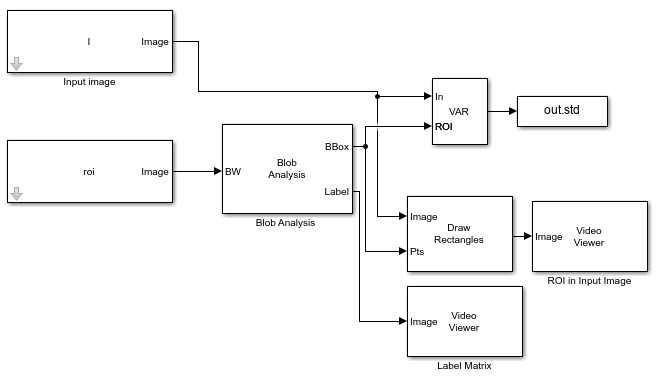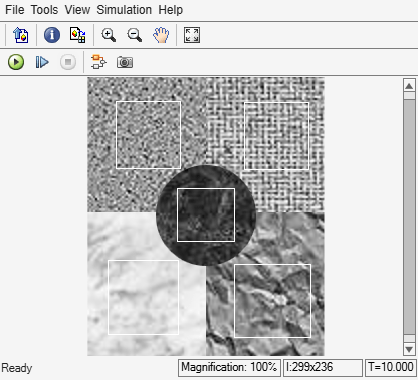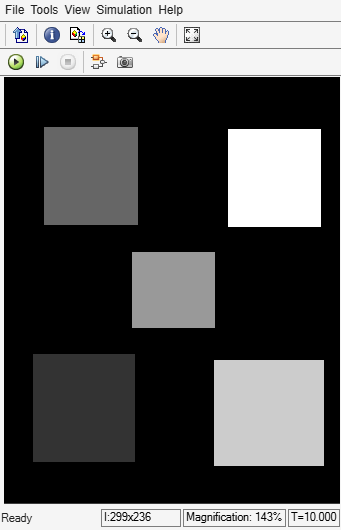# Compute Variance of ROIs

This example shows how to compute the variance of regions of interest (ROIs) in the input image. The input image consists of different texture regions, and a mask image specifies ROIs selected to contain the texture regions.

Read the image into the MATLAB® workspace.

`I = imread("multitextures.png");`

Load the mask image that specifies the ROIs in the input image.

`load("binaryROI.mat")`

### Example Model

```modelname = "ex_blk2DVariance.slx"; open_system(modelname)```The model computes the coordinates for the ROIs by using a Blob Analysis block. The The value of the Maximum number of blobs parameter of the Blob Analysis block is 5, corresponding to the number of ROIs in the image.

The 2-D Variance block computes the variance value for each ROI.

Set these parameters of the 2-D Variance block to the specified value to compute individual statistics for each ROI.

• `Find the variance value over` `-` `Entire input`

• `Enable ROI processing` `- on`

• `ROI type - Rectangles`

• `Output` - `Individual statistics for each ROI`

### Simulate and Display Results

Run the model. The Video Viewer blocks display the input image and the label matrix that corresponds to the selected ROIs. The rectangles overlaid on the input image represent the ROIs for which the model computes the variance.

`output = sim(modelname)````output = Simulink.SimulationOutput: std: [5x1 double] tout: [51x1 double] SimulationMetadata: [1x1 Simulink.SimulationMetadata] ErrorMessage: [0x0 char] ```

The variance value for an ROI indicates the dispersion of the pixel values in that ROI from the mean value in the ROI. Display the variance value for each ROI. The first variance value corresponds to the region with label value `1`, the second variance value corresponds to the region with label value `2`, and so on.

`output.std`
```ans = 5×1 0.0029 0.0145 0.0060 0.0214 0.0265 ```